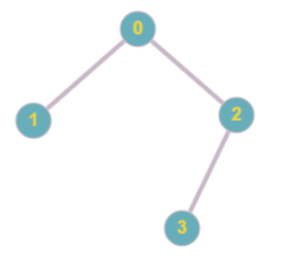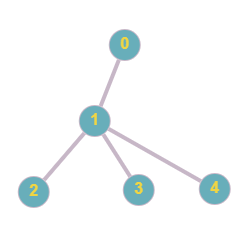Problem title
Difficulty
Avg time to solve

Split Array With the Same Average
Hard
15 mins
Power of Three
Easy
15 mins
The Maze
Moderate
35 mins
Maximum Difference
Easy
15 mins
Range Sum of BST
Easy
20 mins
Equalize Weights
Moderate
15 mins
Synonymous Sentences
Moderate
20 mins
Rabbit Jumping
Easy
20 mins
Stepping Numbers
Moderate
30 mins
Kronecker Product Of Two Matrices
Easy
20 mins

# Ninja and Employees

Difficulty: EASY
Avg. time to solve
20 min
Success Rate
70%

Problem Statement

#### You are given a tree having ‘N’ nodes and ‘N’-1 edges. And an array ‘POINTS’ where POINTS[i] denotes the importance points of employee with ID as i and an integer ‘X’ is given. Print the sum of importance points of employee ‘X’ and all its subordinates.

##### For Example
``````If the given tree:
And POINTS = [3,5,1,4] and X = 2
``````The answer will be 5 (Points of 2 + Points of 3).

##### Input Format:
``````The first line of the input contains an integer, 'T,’ denoting the number of test cases.

The first line of each test case contains a single integer ‘N’ denoting the number of kingdoms.

The next ‘N’-1 lines of each test case contain two integers representing an edge between the given indices.
The next line contains the ‘POINTS’ array.
The next line contains a single integer ‘X’.
``````
##### Output Format:
``````For each test case, print the sum of importance points of Employee ‘X’ and all its subordinates.

Print the output of each test case in a separate line.
``````
##### Note:
``````You do not need to print anything. It has already been taken care of. Just implement the given function.
``````
##### Constraints:
``````1 <= T <= 10
1 <= N <= 10^6.
1 <= POINTS[i] <= 1000
0 <= X < N.

Time limit: 1 sec
``````
##### Sample Input 1:
``````2
4
0 1
0 2
2 3
3 5 1 4
2
5
0 1
1 2
1 3
1 4
1 2 3 4 5
0
``````
##### Sample Output 1:
``````5
15
``````
##### Explanation of sample input 1:
``````For the first test case,
``````The subordinates of Node 2 is 3 only.So the answer will be POINTS + POINTS= 5. Hence,the answer is 5.

``````For the second test case:
````````````The subordinates of Node 0 are Node 1, Node 2, Node 3, and Node 4. So the answer will be the sum of points of all these nodes.Answer will be (POINTS + POINTS + POINTS + POINTS +POINTS  )= 15.
``````
##### Sample Input 2:
``````2
6
0 1
0 5
1 2
1 3
3 4
7 3 3 10 10 9
2
9
0 1
0 4
0 7
1 2
2 3
3 6
4 5
4 8
1 2 8 5 5 6 2 3 4
1
``````
##### Sample Output 2:
``````3
17
``````Console